# Performance Measures for Machine Learning 1 Performance Measures

• Slides: 32Performance Measures for Machine Learning 1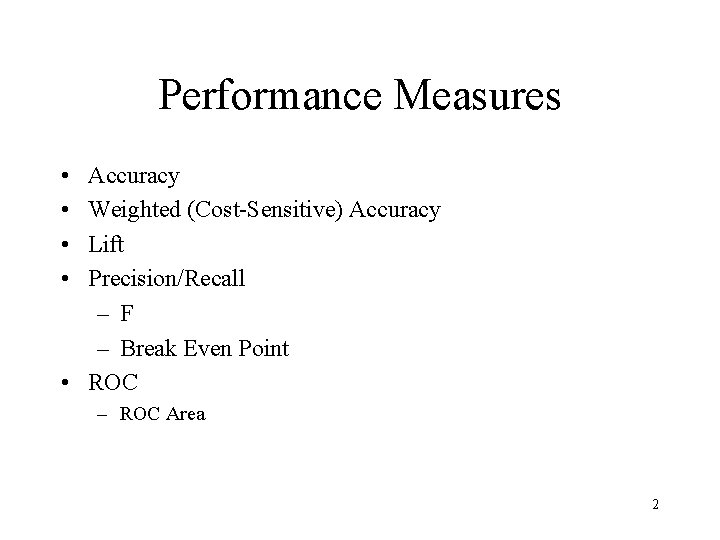Performance Measures • • Accuracy Weighted (Cost-Sensitive) Accuracy Lift Precision/Recall – F – Break Even Point • ROC – ROC Area 2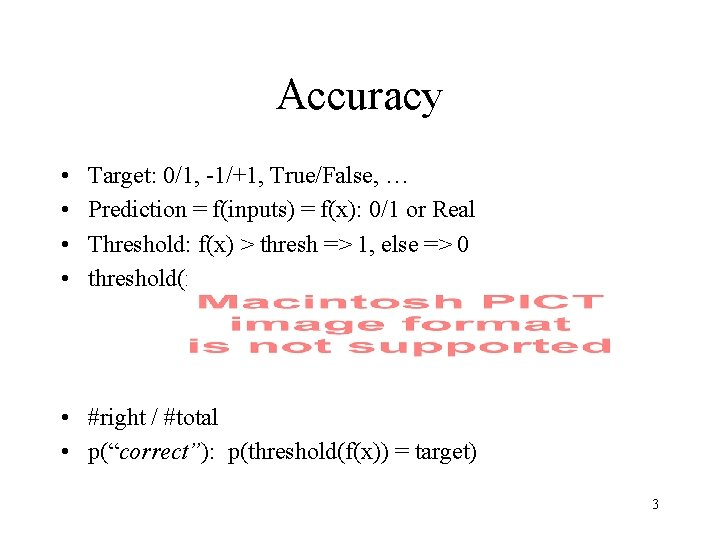Accuracy • • Target: 0/1, -1/+1, True/False, … Prediction = f(inputs) = f(x): 0/1 or Real Threshold: f(x) > thresh => 1, else => 0 threshold(f(x)): 0/1 • #right / #total • p(“correct”): p(threshold(f(x)) = target) 3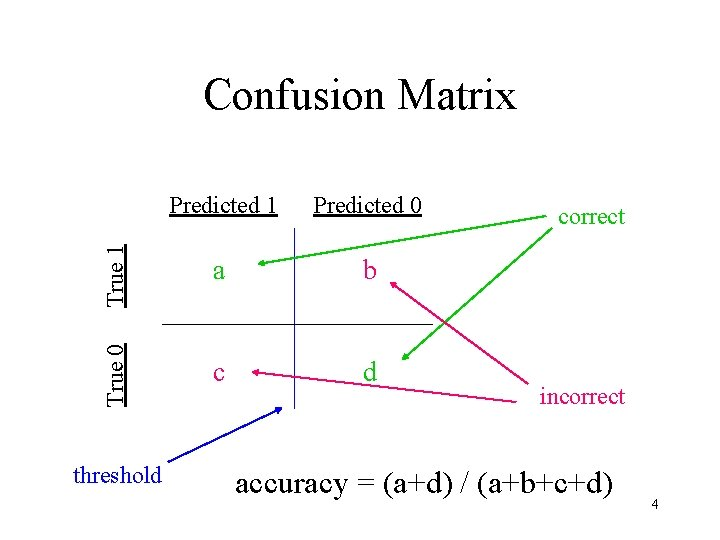Predicted 1 Predicted 0 True 1 a b True 0 Confusion Matrix c d threshold correct incorrect accuracy = (a+d) / (a+b+c+d) 4Prediction Threshold True 1 0 b True 0 Predicted 1 Predicted 0 0 d • threshold > MAX(f(x)) • all cases predicted 0 • (b+d) = total • accuracy = %False = %0’s True 1 a 0 True 0 Predicted 1 Predicted 0 c 0 • threshold < MIN(f(x)) • all cases predicted 1 • (a+c) = total • accuracy = %True = %1’s 5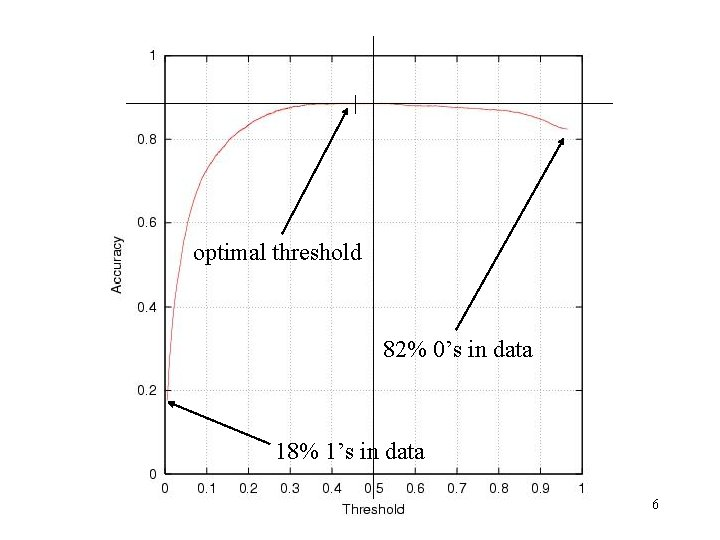optimal threshold 82% 0’s in data 18% 1’s in data 6threshold demo 7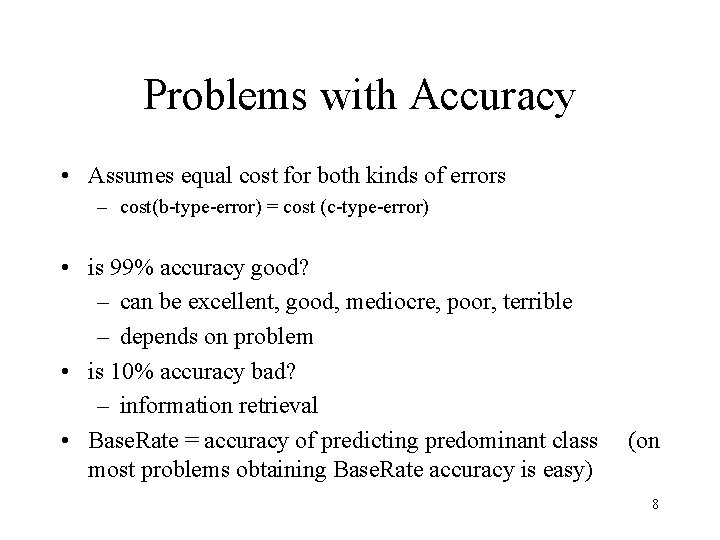Problems with Accuracy • Assumes equal cost for both kinds of errors – cost(b-type-error) = cost (c-type-error) • is 99% accuracy good? – can be excellent, good, mediocre, poor, terrible – depends on problem • is 10% accuracy bad? – information retrieval • Base. Rate = accuracy of predicting predominant class most problems obtaining Base. Rate accuracy is easy) (on 8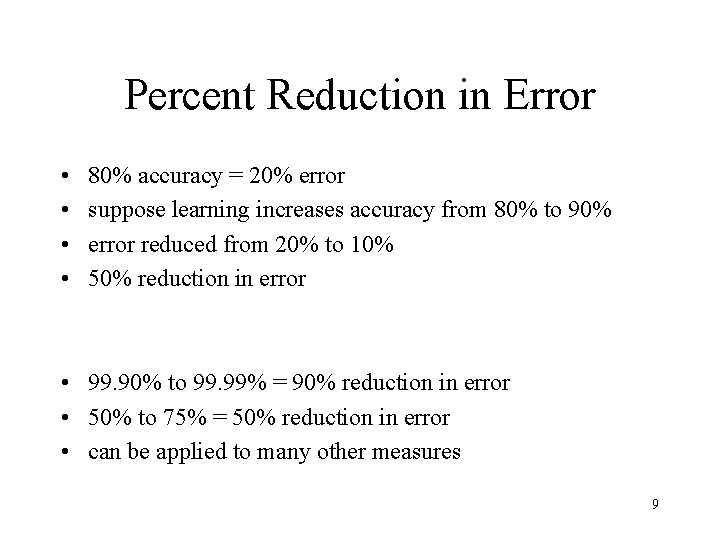Percent Reduction in Error • • 80% accuracy = 20% error suppose learning increases accuracy from 80% to 90% error reduced from 20% to 10% 50% reduction in error • 99. 90% to 99. 99% = 90% reduction in error • 50% to 75% = 50% reduction in error • can be applied to many other measures 9Costs (Error Weights) True 1 Predicted 0 wa wb True 0 Predicted 1 wc wd • Often Wa = Wd = zero and Wb ≠ Wc ≠ zero 101112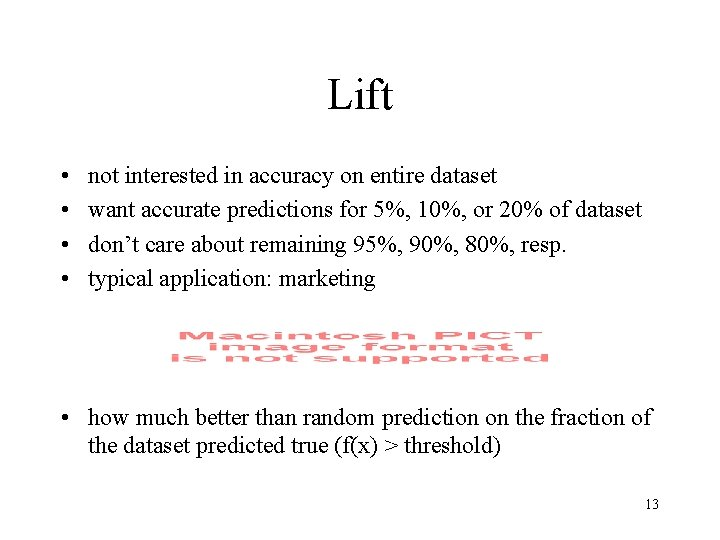Lift • • not interested in accuracy on entire dataset want accurate predictions for 5%, 10%, or 20% of dataset don’t care about remaining 95%, 90%, 80%, resp. typical application: marketing • how much better than random prediction on the fraction of the dataset predicted true (f(x) > threshold) 13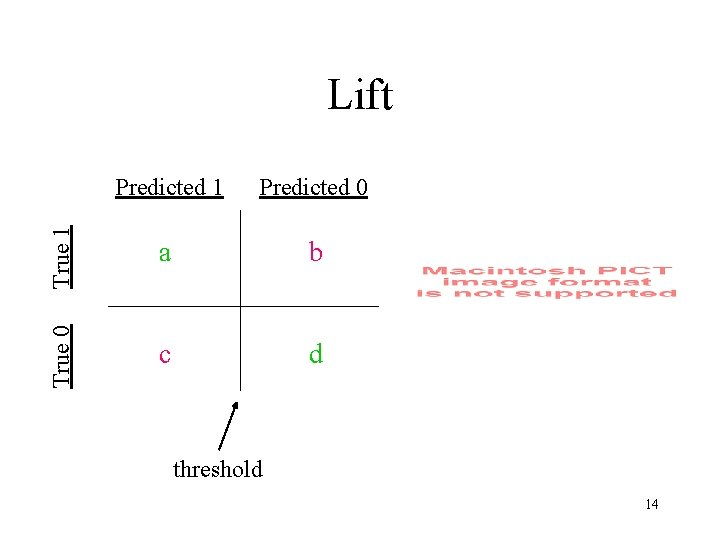Predicted 1 Predicted 0 True 1 a b True 0 Lift c d threshold 14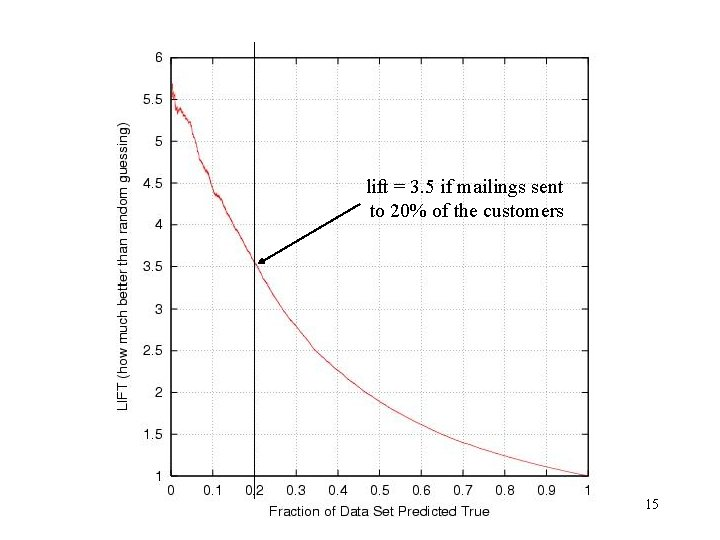lift = 3. 5 if mailings sent to 20% of the customers 15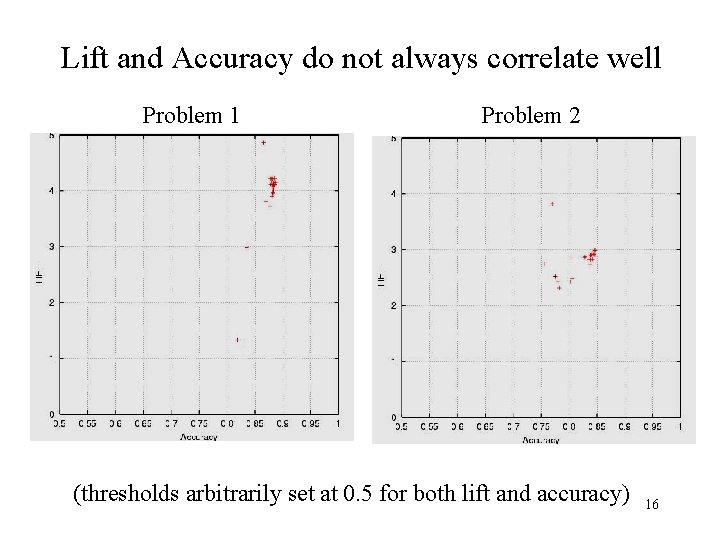Lift and Accuracy do not always correlate well Problem 1 Problem 2 (thresholds arbitrarily set at 0. 5 for both lift and accuracy) 16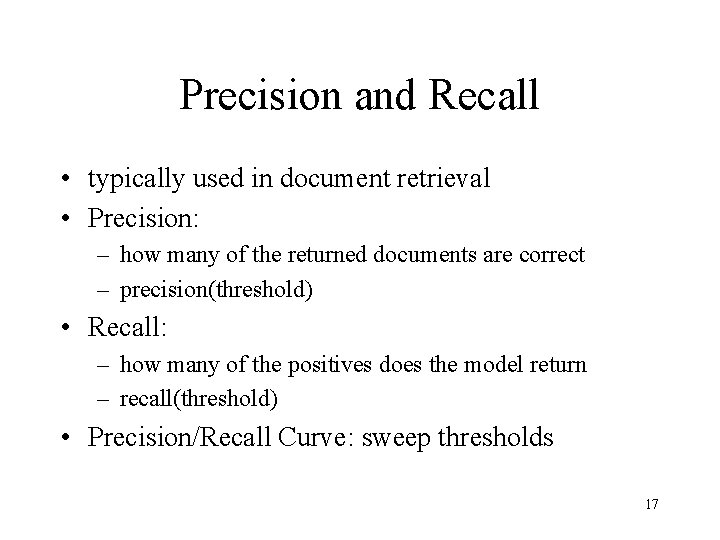Precision and Recall • typically used in document retrieval • Precision: – how many of the returned documents are correct – precision(threshold) • Recall: – how many of the positives does the model return – recall(threshold) • Precision/Recall Curve: sweep thresholds 17Predicted 1 Predicted 0 True 1 a b True 0 Precision/Recall c d threshold 1819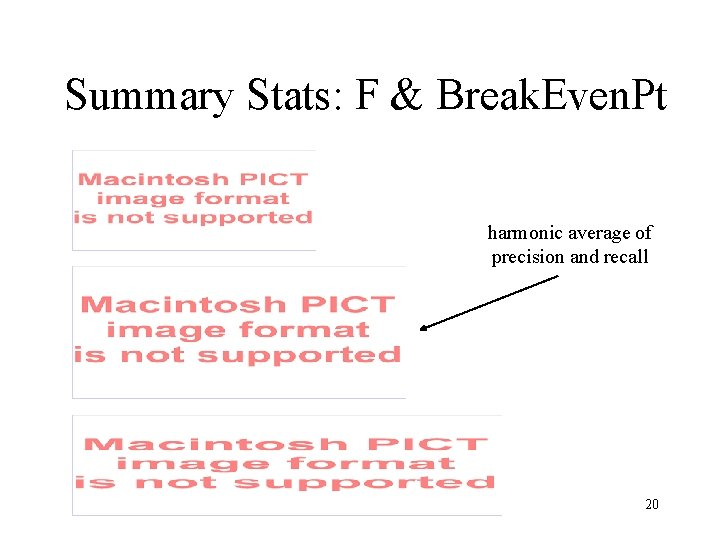Summary Stats: F & Break. Even. Pt harmonic average of precision and recall 20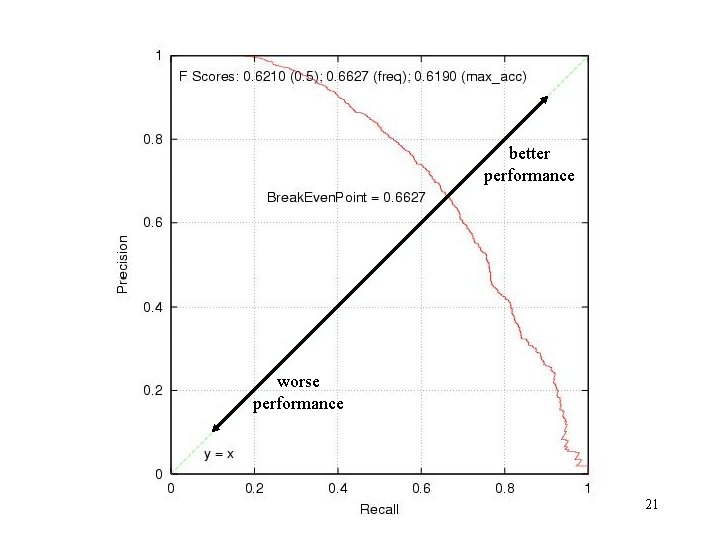better performance worse performance 21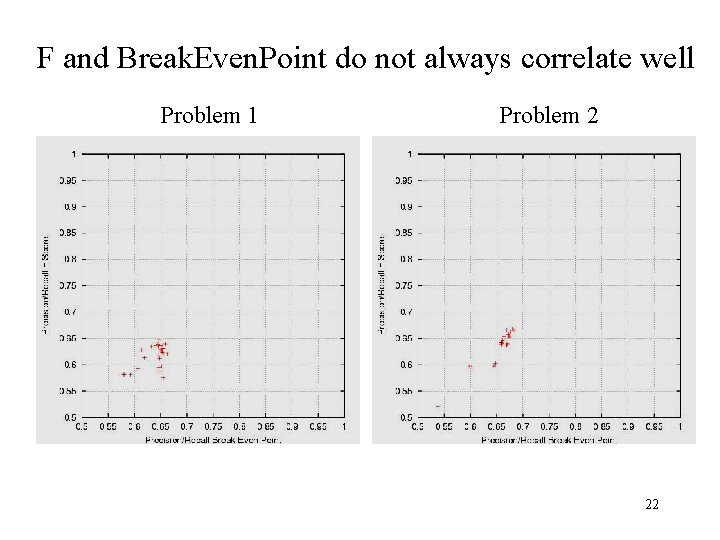F and Break. Even. Point do not always correlate well Problem 1 Problem 2 22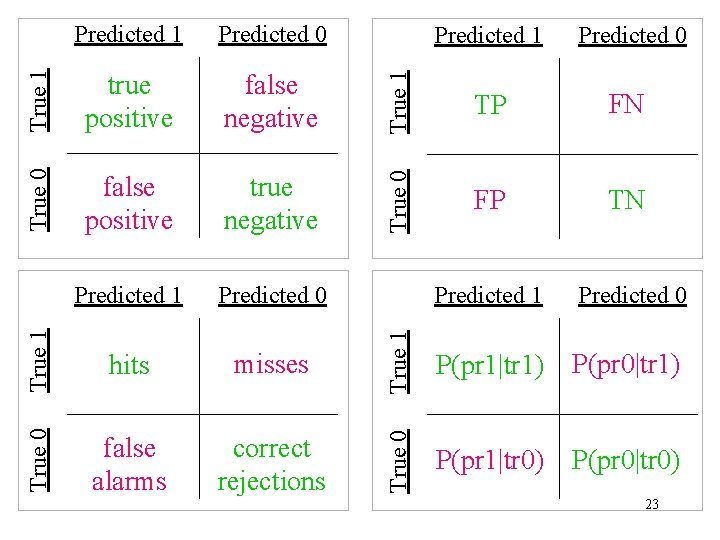True 0 false positive true negative Predicted 1 Predicted 0 True 1 hits misses True 0 false alarms correct rejections True 1 false negative Predicted 0 TP FN True 0 True 1 true positive Predicted 1 FP TN Predicted 1 Predicted 0 True 1 Predicted 0 P(pr 1|tr 1) P(pr 0|tr 1) True 0 Predicted 1 P(pr 1|tr 0) P(pr 0|tr 0) 23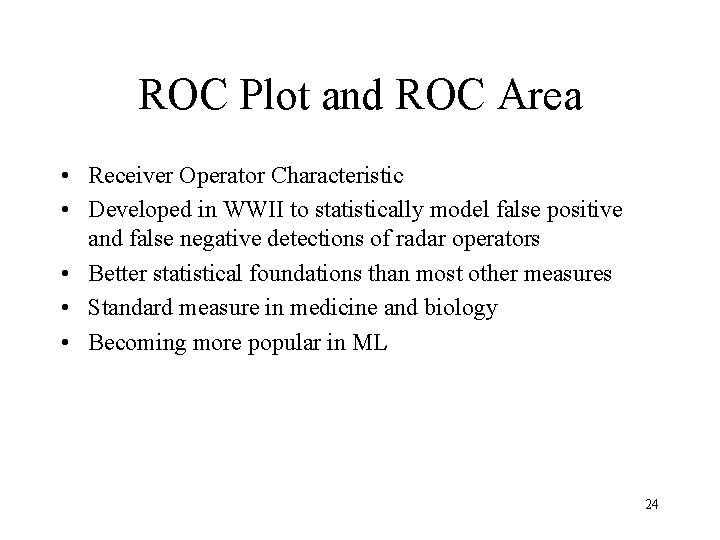ROC Plot and ROC Area • Receiver Operator Characteristic • Developed in WWII to statistically model false positive and false negative detections of radar operators • Better statistical foundations than most other measures • Standard measure in medicine and biology • Becoming more popular in ML 24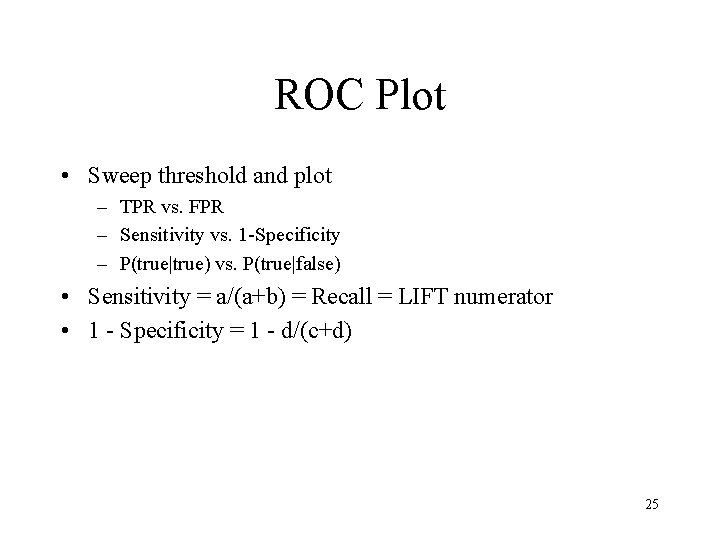ROC Plot • Sweep threshold and plot – TPR vs. FPR – Sensitivity vs. 1 -Specificity – P(true|true) vs. P(true|false) • Sensitivity = a/(a+b) = Recall = LIFT numerator • 1 - Specificity = 1 - d/(c+d) 25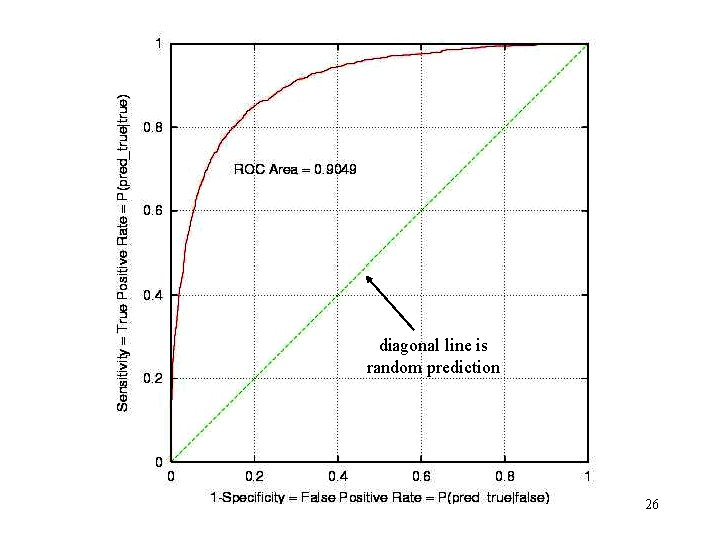diagonal line is random prediction 26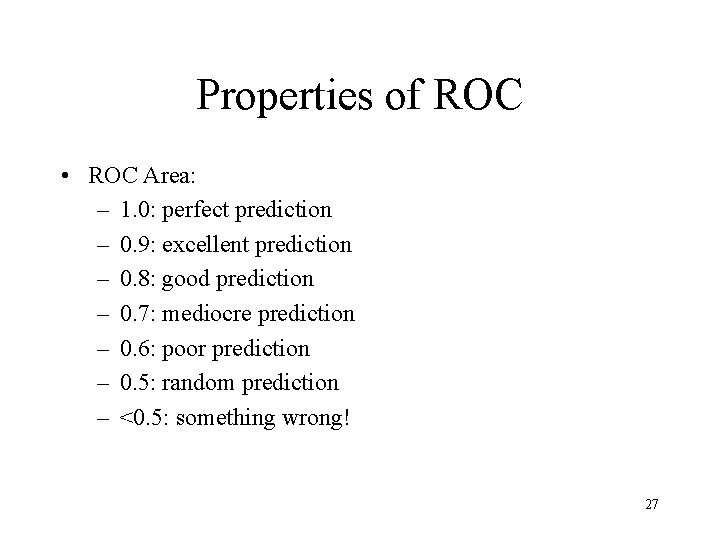Properties of ROC • ROC Area: – 1. 0: perfect prediction – 0. 9: excellent prediction – 0. 8: good prediction – 0. 7: mediocre prediction – 0. 6: poor prediction – 0. 5: random prediction – <0. 5: something wrong! 27Properties of ROC • Slope is non-increasing • Each point on ROC represents different tradeoff (cost ratio) between false positives and false negatives • Slope of line tangent to curve defines the cost ratio • ROC Area represents performance averaged over all possible cost ratios • If two ROC curves do not intersect, one method dominates the other • If two ROC curves intersect, one method is better for some cost ratios, and other method is better for other cost ratios 28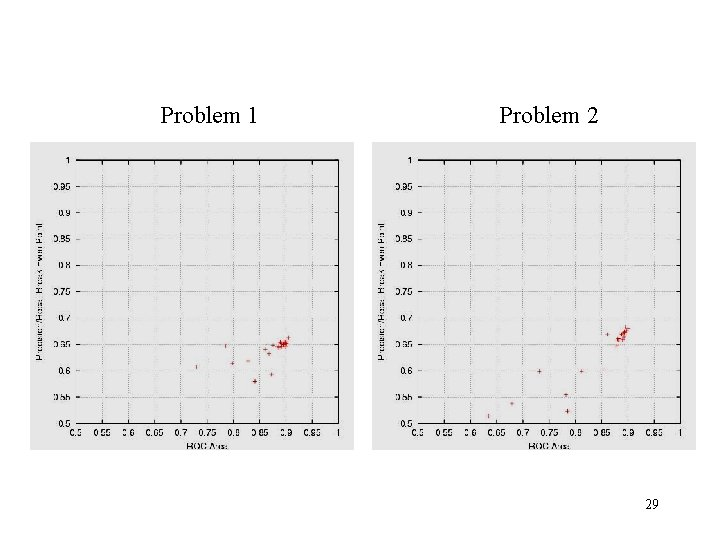Problem 1 Problem 2 29Problem 1 Problem 2 30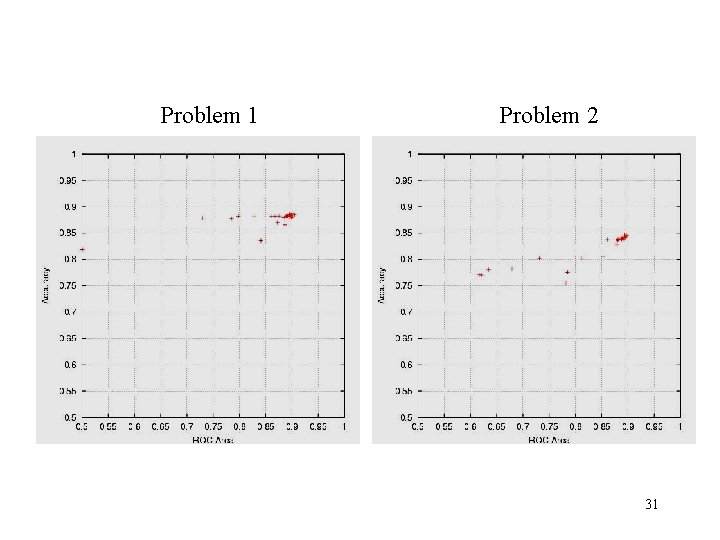Problem 1 Problem 2 31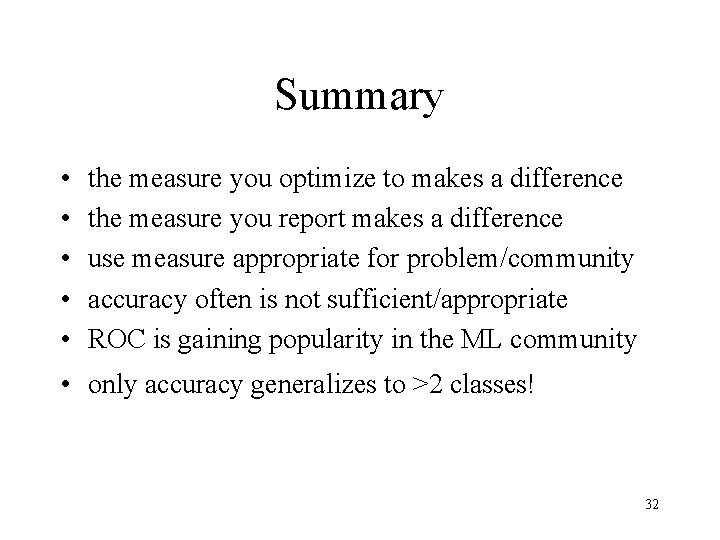Summary • • • the measure you optimize to makes a difference the measure you report makes a difference use measure appropriate for problem/community accuracy often is not sufficient/appropriate ROC is gaining popularity in the ML community • only accuracy generalizes to >2 classes! 32